# Oops...

This website is made for modern browsers. You are seeing this, because your browser is missing a feature or two. Please install the latest update or switch to a modern browser. See you soon.

# Split curves

This how-to guide will recap various methods to split curves at intersection events and how to get the remaining segments. The how-to Divide curves is focusing on a segmentation with a regular or pattern-based logic. To select the curves behind or before the intersecting shape and how to remove them, see Trim curves.

For the general purpose splitting of curves, we can use the component Shatter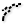Shatter (Shatter)
Curve  >  Division  >  Shatter
Shatter a curve into segments.
Inputs
Curve (C)Curve to trim
Parameters (t)Parameters to split at
Outputs
Segments (S)Shattered remains
. Input C takes the curves to split and input t is asking for the curve parameter to split at. The parameter t is therefore no point, but relates the distance from the curve’s start to the desired mark. Therefore, before we can do any splitting, we have to compute the necessary values for t.

In simple cases, there is no need to calculate the parameter to split at, because we can directly set it. For example, if we would like to split a line or regular curve in the middle, we can reparameterize the curve and set t to 0.5.

Note that for complex curves, like Nurbs curves, the midpoint might not be at parameter 0.5.
Note that for complex curves, like Nurbs curves, the midpoint might not be at parameter 0.5.

If you need to split polylines at the kinks, you can use the component Explode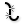Explode (Explode)
Curve  >  Util  >  Explode
Explode a curve into smaller segments.
Inputs
Curve (C)Curve to explode
Recursive (R)Recursive decomposition until all segments are atomic
Outputs
Segments (S)Exploded segments that make up the base curve
Vertices (V)Vertices of the exploded segments
.

## Calculate curve parameters for splitting

As said, before we can do the actual splitting, we have to compute the parameters to split at. The ribbon tab Intersect contains components for different intersection events. Some components will directly output the sought-for curve parameters t, while others just emit the intersection point P and we have to retrieve the splitting parameter t with an extra step.

### Find parameters to split at a component’s output (two distinct sets of curves)

Given are two sets of curves: Set A contains vertical curves and set B consists of one horizontal curve. We can solve the intersection of the two sets with the component Curve | CurveCurve | Curve (CCX)
Intersect  >  Physical  >  Curve | Curve
Solve intersection events for two curves.
Inputs
Curve A (A)First curve
Curve B (B)Second curve
Outputs
Points (P)Intersection events
Params A (tA)Parameters on first curve
Params B (tB)Parameters on second curve
. Beside the actual intersection points P, we also get the curve parameters for each set, tA for set A and tB for set B. Now, we connect the curves from set A and the curve parameters tA to a ShatterShatter (Shatter)
Curve  >  Division  >  Shatter
Shatter a curve into segments.
Inputs
Curve (C)Curve to trim
Parameters (t)Parameters to split at
Outputs
Segments (S)Shattered remains
component. We also have to graft to adjust the data structure
at input C.

This procedure will split the curves in Set A. If we wish to also split set B, we have to use another ShatterShatter (Shatter)
Curve  >  Division  >  Shatter
Shatter a curve into segments.
Inputs
Curve (C)Curve to trim
Parameters (t)Parameters to split at
Outputs
Segments (S)Shattered remains
component in a corresponding way.

Next, let us assume that there is another horizontal curve in set B (the wire has now switched to two parallel lines). To ensure that every curve from one set is compared with each curve from the other set, we have to adjust the data structure and graft either of the two input grips of Curve | CurveCurve | Curve (CCX)
Intersect  >  Physical  >  Curve | Curve
Solve intersection events for two curves.
Inputs
Curve A (A)First curve
Curve B (B)Second curve
Outputs
Points (P)Intersection events
Params A (tA)Parameters on first curve
Params B (tB)Parameters on second curve
. After solving the intersection, we have to reduce the data tree by one level, which is done with Trim TreeTrim Tree (Trim)
Sets  >  Tree  >  Trim Tree
Reduce the complexity of a tree by merging the outermost branches.
Inputs
Tree (T)Data tree to flatten
Depth (D)Number of outermost branches to merge
Outputs
Tree (T)Trimmed data tree
. Then we can use ShatterShatter (Shatter)
Curve  >  Division  >  Shatter
Shatter a curve into segments.
Inputs
Curve (C)Curve to trim
Parameters (t)Parameters to split at
Outputs
Segments (S)Shattered remains
for splitting the curves.

A similar procedure can be used with the components Line | Line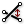Line | Line (LLX)
Intersect  >  Mathematical  >  Line | Line
Solve intersection events for two lines.
Inputs
Line 1 (A)First line for intersection
Line 2 (B)Second line for intersection
Outputs
Param A (tA)Parameter on line A
Param B (tB)Parameter on line B
Point A (pA)Point on line A
Point B (pB)Point on line B
, Curve | Line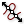Curve | Line (CLX)
Intersect  >  Mathematical  >  Curve | Line
Solve intersection events for a curve and a line.
Inputs
Curve (C)Curve to intersect
Line (L)Line to intersect with
Outputs
Points (P)Intersection events
Params (t)Parameters on curve
Count (N)Number of intersection events
, Curve | Self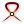Curve | Self (CX)
Intersect  >  Physical  >  Curve | Self
Solve all self intersection events for a curve.
Inputs
Curve (C)Curve for self-intersections
Outputs
Points (P)Intersection events
Params (t)Parameters on curve
and a few others in the ribbon tab Intersect.

### Find parameters to split at a component’s output (unsorted bundle of curves)

If all curves are bundled in a single list and there is no distinct set to solve the intersection event for, we can use Multiple Curves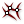Multiple Curves (MCX)
Intersect  >  Physical  >  Multiple Curves
Solve intersection events for multiple curves.
Inputs
Curves (C)Curves to intersect
Outputs
Points (P)Intersection events
Index A (iA)Index of first intersection curve
Index B (iB)Index of second intersection curve
Param A (tA)Parameter on first curve
Param B (tB)Parameter on second curve
to compare every curve with every other. Even though we get the curve parameters for each curve (tA and tB) there is no simple output that gives us the corresponding curves: The outputs iA and iB state the indices of the curves that each intersection events belongs to, but we have to manually sort the curves and group all intersections that each curve is subject to.

To proceed, we will first bundle our outputs with Merge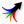Merge (Merge)
Sets  >  Tree  >  Merge
Merge a bunch of data streams
Inputs
Data 1 (D1)Data stream 1
Data 2 (D2)Data stream 2
Outputs
Result (R)Result of merge
, so that both lists correspond to each other. Then we use Create Set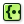Create Set (CSet)
Sets  >  Sets  >  Create Set
Creates the valid set from a list of items (a valid set only contains distinct elements).
Inputs
List (L)List of data.
Outputs
Set (S)A set of all the distincts values in L
Map (M)An index map from original indices to set indices
and Member Index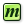Member Index (MIndex)
Sets  >  Sets  >  Member Index
Find the occurences of a specific member in a set.
Inputs
Set (S)Set to operate on.
Member (M)Member to search for.
Outputs
Index (I)Indices of member.
Count (N)Number of occurences of the member.
to create a data tree in which each branch holds a unique curve index. The number of index repetitions in each branch is given by the number of intersection events for each curve.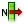List Item (Item)
Sets  >  List  >  List Item
Retrieve a specific item from a list.
Inputs
List (L)Base list
Index (i)Item index
Wrap (W)Wrap index to list bounds
Outputs
Item (i)Item at {i'}
to retrieve the curves that we want to split from output S of Create Set. We also need another List ItemList Item (Item)
Sets  >  List  >  List Item
Retrieve a specific item from a list.
Inputs
List (L)Base list
Index (i)Item index
Wrap (W)Wrap index to list bounds
Outputs
Item (i)Item at {i'}
to group the curve parameters for each curve. Finally, we use ShatterShatter (Shatter)
Curve  >  Division  >  Shatter
Shatter a curve into segments.
Inputs
Curve (C)Curve to trim
Parameters (t)Parameters to split at
Outputs
Segments (S)Shattered remains
to do the splitting.

Do note that curves which did not have an intersection event are not part of the output. The component Combine Data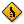Combine Data (Combine)
Sets  >  List  >  Combine Data
Combine non-null items out of several inputs
Inputs
Input 0 (0)Data to combine
Input 1 (1)Data to combine
Outputs
Result (R)Resulting data with as few nulls as possible
Index (I)Index of input that was copied into result
can be used to merge all curves into a data tree, as it is done in the next chapter.

### Compute parameters to split with intersection points

For this example, we take the unsorted bundle of curves from the previous chapter and again, we use Multiple CurvesMultiple Curves (MCX)
Intersect  >  Physical  >  Multiple Curves
Solve intersection events for multiple curves.
Inputs
Curves (C)Curves to intersect
Outputs
Points (P)Intersection events
Index A (iA)Index of first intersection curve
Index B (iB)Index of second intersection curve
Param A (tA)Parameter on first curve
Param B (tB)Parameter on second curve
to solve their intersection events. Unlike before, we are not using the curve parameters to proceed but instead the intersection points at output P. In order to use ShatterShatter (Shatter)
Curve  >  Division  >  Shatter
Shatter a curve into segments.
Inputs
Curve (C)Curve to trim
Parameters (t)Parameters to split at
Outputs
Segments (S)Shattered remains
, we have to compute the curve parameters with a different method: Curve Closest Point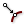Curve Closest Point (Crv CP)
Curve  >  Analysis  >  Curve Closest Point
Find the closest point on a curve.
Inputs
Point (P)Point to project onto curve
Curve (C)Curve to project onto
Outputs
Point (P)Point on the curve closest to the base point
Parameter (t)Parameter on curve domain of closest point
Distance (D)Distance between base point and curve
will generate the closest point on a curve in relation to a given point.

After grafting input C of Curve Closest PointCurve Closest Point (Crv CP)
Curve  >  Analysis  >  Curve Closest Point
Find the closest point on a curve.
Inputs
Point (P)Point to project onto curve
Curve (C)Curve to project onto
Outputs
Point (P)Point on the curve closest to the base point
Parameter (t)Parameter on curve domain of closest point
Distance (D)Distance between base point and curve
, we compute the distance of every intersection point to every curve. But we are only interested in the points that are actually on the curves. To get them, we use Smaller Than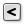Smaller Than (Smaller)
Maths  >  Operators  >  Smaller Than
Smaller than (or equal to)
Inputs
First Number (A)Number to test
Second Number (B)Number to test against
Outputs
Smaller than (<)True if A < B
… or Equal to (<=)True if A <= B
and Dispatch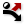Dispatch (Dispatch)
Sets  >  List  >  Dispatch
Dispatch the items in a list into two target lists.
Inputs
List (L)List to filter
Dispatch pattern (P)Dispatch pattern
Outputs
List A (A)Dispatch target for True values
List B (B)Dispatch target for False values
to select the desired curve parameters t for points that have no distance D to the curve. Instead of 0 we use 1e-10 to account for software-related inaccuracies.

ShatterShatter (Shatter)
Curve  >  Division  >  Shatter
Shatter a curve into segments.
Inputs
Curve (C)Curve to trim
Parameters (t)Parameters to split at
Outputs
Segments (S)Shattered remains
will split our curves into segments. But, as mentioned before, curves that had no intersection event will not be part of the output. To create a data tree that contains all curves, we can use Combine DataCombine Data (Combine)
Sets  >  List  >  Combine Data
Combine non-null items out of several inputs
Inputs
Input 0 (0)Data to combine
Input 1 (1)Data to combine
Outputs
Result (R)Resulting data with as few nulls as possible
Index (I)Index of input that was copied into result
. This is useful if we would like to continue with a trimming action.

A similar procedure can be used with the components Brep | Curve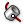Brep | Curve (BCX)
Intersect  >  Physical  >  Brep | Curve
Solve intersection events for a Brep and a curve.
Inputs
Brep (B)Base Brep
Curve (C)Intersection curve
Outputs
Curves (C)Intersection overlap curves
Points (P)Intersection points
, Surface | Curve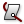Surface | Curve (SCX)
Intersect  >  Physical  >  Surface | Curve
Solve intersection events for a surface and a curve.
Inputs
Surface (S)Base surface
Curve (C)Intersection curve
Outputs
Curves (C)Intersection overlap curves
Points (P)Intersection points
UV Points (uv)Surface {uv} coordinates at intersection events
Normals (N)Surface normal vector at intersection events
Parameters (t)Curve parameter at intersection events
Tangents (T)Curve tangent vector at intersection events
, Curve | Plane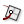Curve | Plane (PCX)
Intersect  >  Mathematical  >  Curve | Plane
Solve intersection events for a curve and a plane.
Inputs
Curve (C)Base curve
Plane (P)Intersection plane
Outputs
Points (P)Intersection events
Params C (t)Parameters {t} on curve
Params P (uv)Parameters {uv} on plane
, Mesh | Curve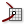Mesh | Curve (MCX)
Intersect  >  Physical  >  Mesh | Curve
Mesh Curve intersection
Inputs
Mesh (M)Mesh to intersect
Curve (C)Curve to intersect with
Outputs
Points (X)Intersection points
Faces (F)Intersection face index for each point
and others in the ribbon tab Intersect, which do not emit a curve parameter t but intersection points.

This page is open source. Edit it on GitHub or see how you can contribute.